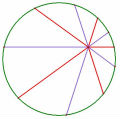# 101 宜蘭高中

## 101 宜蘭高中

1. 雙曲線與直線 x+y=8 相切 , 焦點 (0,4 ),(10,0) , 求雙曲線正焦弦長 ?

2. (1*2/2*3)+(2*2^2/3*4)+(3*2^3/4*5)+......+(10*2^10/11*12) 的最簡分數?

(100中科是 考相乘 , 改成相加, 我用暴力解, 有否其他做法 ? )

3. a,b 為實數 , 求根號 [(a-6)^2+4] + 根號 [a^2+b^2] + 根號 [ (b-3)^2+16 ] 的最小值 ?

(題目我忘了, 化簡成如上, 請問要如何判斷最小值 ? )

4. 五位數, 各位數字和為12 , 請問有幾個五數字符合?

[ 本帖最後由 mandy 於 2012-6-8 04:54 PM 編輯 ]

TOP

##### 引用:

1. 雙曲線與直線 x+y=8 相切 , 焦點 (0,4 ),(10,0) , 求雙曲線正焦弦長 ?

K為F' 以x+y=8為對稱軸的對稱點,則K(4,8)

PF-PF' =PF-PK=KF=10=2a ,a=5
2c=FF'=2*29^0.5 ,c=29^0.5
b^2=c^2-a^2=29-25=4 ,b=2

TOP

## 4. 五位數, 各位數字和為12 , 請問有幾個五數字符合?

4. 五位數, 各位數字和為12 , 請問有幾個五數字符合?

a+b+c+d+e=12,  a>1 ,  a,b,c,d,e<10

(a-1)+b+c+d+e=11

=H(5,11)-5-C(5,2)
=1365-5-20=1340

TOP

## 填充四

=C(15,11)-4*C(5,1)-C(6,2)
=1365-20-15
=1330

TOP

 老王 老王發私訊 加為好友 目前離線 5# 大 中 小 發表於 2012-6-8 22:00  只看該作者 $$\displaystyle \sum _{k=1}^{10} \frac{k \times 2^k}{(k+1)(k+2)}$$ $$\displaystyle =\sum _{k=1}^{10} k \times 2^k \times (\frac{1}{k+1}-\frac{1}{k+2})$$ $$\displaystyle =1+\sum _{k=1}^9 \frac{1}{k+2}[(k+1) \times 2^{k+1}-k \times 2^k]-\frac{10 \times 2^{10}}{12}$$ $$\displaystyle =1+\sum _{k=1}^9 \frac{1}{k+2} \times 2^k \times (2k+2-k)-\frac{5 \times 2^9}{3}$$ $$\displaystyle =1+2+4+ \cdots +512-\frac{2560}{3}$$ $$\displaystyle =\frac{509}{3}$$ 名豈文章著官應老病休飄飄何所似Essential isolated singularity UID261 帖子308 閱讀權限10 上線時間943 小時 註冊時間2009-5-14 最後登入2014-3-17  查看詳細資料 TOP
##### 引用:

$$\displaystyle \sum _{k=1}^{10} \frac{k \times 2^k}{(k+1)(k+2)}$$

$$\displaystyle =\sum _{k=1}^{10} k \times 2^k \times (\frac{1}{k+1}-\frac{1}{k+2})$$ ...

TOP

TOP

## 回復 6# weiye 的帖子

$$\ P(6,-2),A(a,0),B(0,b),Q(-4,3)$$

TOP

## 回復 9# 老王 的帖子

TOP

 mandy發私訊 加為好友 目前離線 10# 大 中 小 發表於 2012-6-9 08:39  只看該作者 請問 ５.　有一比賽, 有5位裁判，裁判給分的方式只有3分、4分、5分, 已知某ㄧ選手得20分, 試問裁判給選手得分的情形有______種 。 UID318 帖子158 閱讀權限10 上線時間188 小時 註冊時間2009-7-17 最後登入2016-1-18  查看詳細資料 TOP
﻿